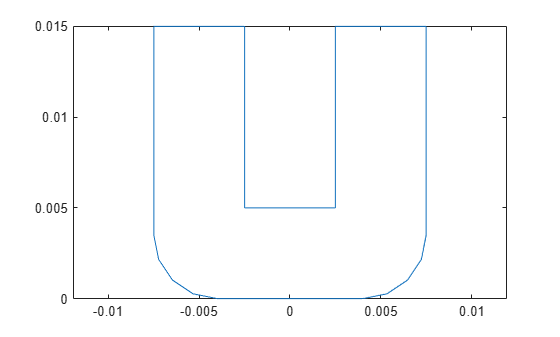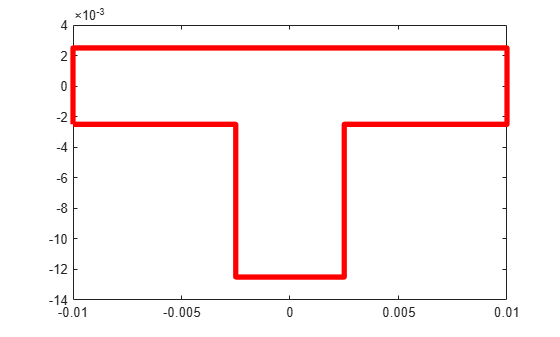# plot

Plot boundary of RF PCB shape

## Syntax

``plot(shape)``
``plot(shape,Name,Value)``
``p = plot(___)``

## Description

example

````plot(shape)` plots the boundary of the shape.```

example

````plot(shape,Name,Value)` specifies the line properties using one or more name-value arguments.Example: `plot(shape,Color="r",LineWidth=2)` plots the boundary of the shape as a red line with a width of 2 pixels.```

example

````p = plot(___)` returns the line object. Use `p` to modify properties of the line after it is created.```

## Examples

collapse all

Create a curved U-bend shape.

`ubend = ubendCurved;`

Plot the shape.

`plot(ubend)`Create a tee trace shape.

`trace = traceTee;`

Plot the shape using a red line of width 4 pixels.

`plot(trace,LineWidth=4,Color="r")`## Input Arguments

collapse all

RF PCB shape created using custom elements and shape objects of RF PCB Toolbox™, specified as an object.

Example: `shape = bendCurved;` specifies the shape as a `bendCurved` object.

## Version History

Introduced in R2021b